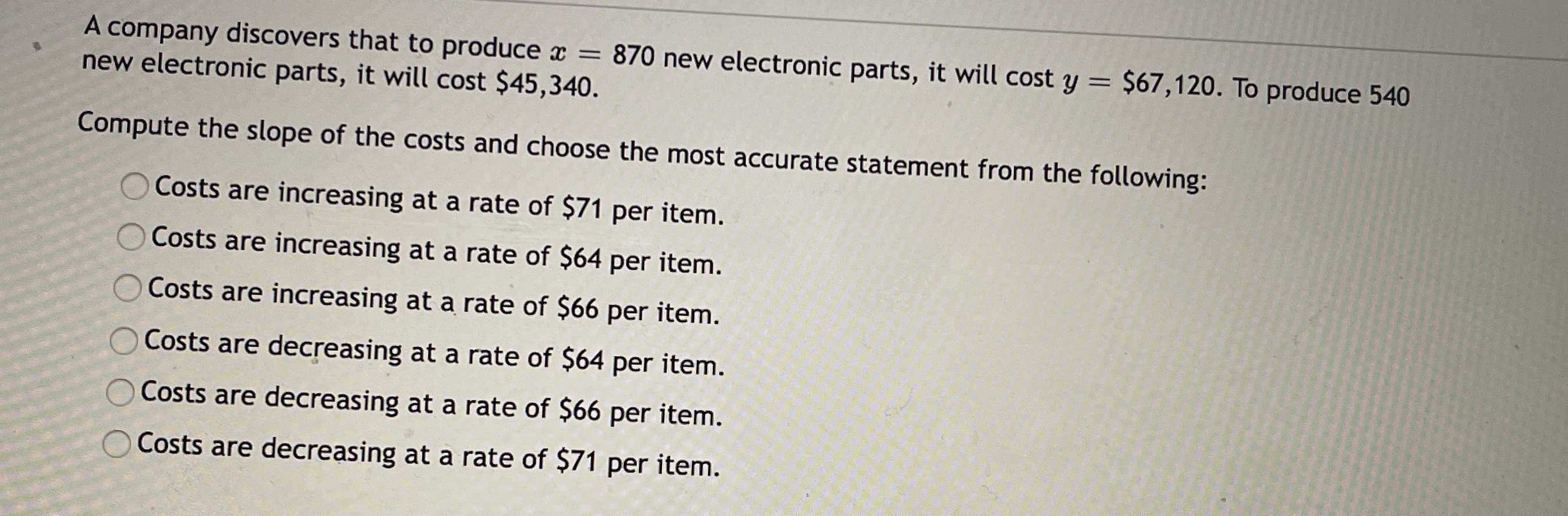### ¿Todavía tienes preguntas de matemáticas?

Pregunte a nuestros tutores expertos
Algebra
PreguntaA company discovers that to produce $$x = 870$$ new electronic parts, it will cost $$y = \ 67,120 .$$ To produce $$540$$ new electronic parts, it will cost $$\ 45,340 .$$

Compute the slope of the costs and choose the most accurate statement from the following:

Costs are increasing at a rate of $$\ 71$$ per item.

Costs are increasing at a rate of $$\ 64$$ per item.

Costs are increasing at a rate of $$\ 66$$ per item.

Costs are decreasing at a rate of $$\ 64$$ per item.

Costs are decreasing at a rate of $$\ 66$$ per item.

Costs are decreasing at a rate of $$\ 71$$ per item.

Costs are increasing at a rate of $$\ 66$$ per item.